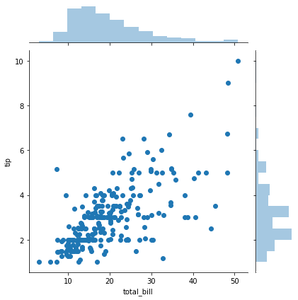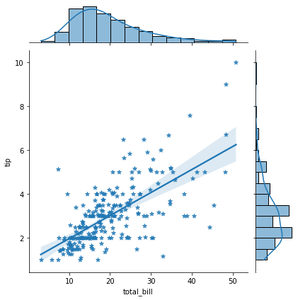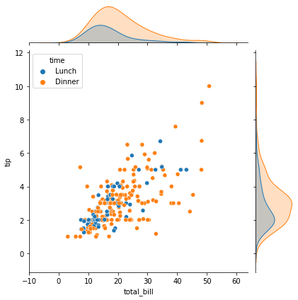Open In App
Related Articles
• Write an Interview Experience

# Scatter Plot with Marginal Histograms in Python with Seaborn

Prerequisites: Seaborn

Scatter Plot with Marginal Histograms is basically a joint distribution plot with the marginal distributions of the two variables. In data visualization, we often plot the joint behavior of two random variables (bi-variate distribution) or any number of random variables. But if data is too large, overlapping can be an issue. Hence, to distinguish between variables it is useful to have the probability distribution of each variable on the side along with the joint plot. This individual probability distribution of a random variable is referred to as its marginal probability distribution.

In seaborn, this is facilitated with jointplot(). It represents the bi-variate distribution using scatterplot() and the marginal distributions using histplot()

### Approach

• Import seaborn library
• Use jointplot() on variables of your dataset

Example 1:

## Python3

 `# importing and creating alias for seaborn``import` `seaborn as sns`` ` `# loading tips dataset``tips ``=` `sns.load_dataset(``"tips"``)`` ` `# plotting scatterplot with histograms for features total bill and tip.``sns.jointplot(data``=``tips, x``=``"total_bill"``, y``=``"tip"``)`

Output :

<seaborn.axisgrid.JointGrid at 0x26203152688>jointplot_with_histograms

Example 2: Using kind=”reg” attribute you can add a linear regression fit and univariate KDE curves.

## Python3

 `import` `seaborn as sns`` ` `tips ``=` `sns.load_dataset(``"tips"``)`` ` `# here "*" is used as a marker for scatterplot``sns.jointplot(data``=``tips, x``=``"total_bill"``, y``=``"tip"``, kind``=``"reg"``, marker``=``"*"``)`

Output :scatterplot with a linear regression fit

Example3: To add conditional colors to the scatterplot you can use hue attribute but it draws separate density curves (using kdeplot()) on the marginal axes.

## Python3

 `import` `seaborn as sns`` ` `tips ``=` `sns.load_dataset(``"tips"``)`` ` `sns.jointplot(data``=``tips, x``=``"total_bill"``, y``=``"tip"``, hue``=``"time"``)`

Output :scatterplot3